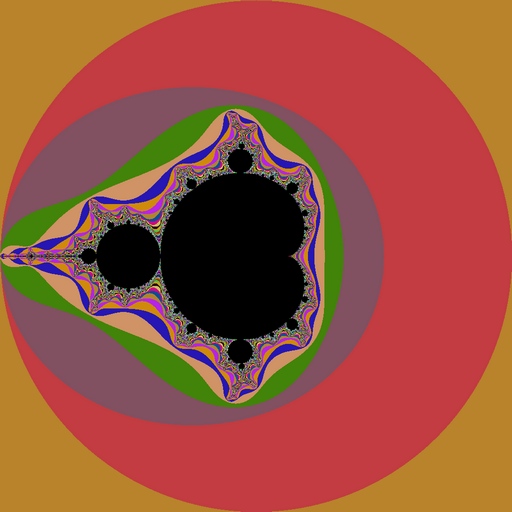### Mandelbrot with HybridizerWe describe here the implementation of Mandelbrot fractal image generation using Hybridizer. The language of choice is C#, and implementation is done using 32-bits precision arithmetic. Mandelbrot set is the set of values c for which the sequence: remains bounded in the complex plane. It happens an equivalent definition is: That means that while calculating […]

Tags: ,